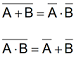# Engineering Mathematics Two Laws and Theorems of De_Morgans

An online De_Morgans Law printable

# De_Morgan’s theorems

DeMorgans Theorems are two additional simplification techniques that can be used to simplify Boolean expressions.

Again, the simpler the Boolean expression the simpler the resulting the Boolean expression, the simpler the resulting logic.The following truth tables prove DeMorgans laws.

INPUT OUTPUT 1 OUTPUT 2
A B NOT (A AND B) (NOT A) OR (NOT B)
0 0 1 1
0 1 1 1
1 0 1 1
1 1 0 0
INPUT OUTPUT 1 OUTPUT 2
A B NOT (A OR B) (NOT A) AND (NOT B)
0 0 1 1
0 1 0 0
1 0 0 0
1 1 0 0

# Definition of De Morgan’s Laws:

The complement of the union of two sets is equal to the intersection of their complements and the complement of the intersection of 2 sets is equal to the union of their complements.These are called De Morgan’s laws.

For any two finite sets A and B;

(i) (A U B)’ = A’ ∩ B’ (which is a De Morgans law of union).

(ii) (A ∩ B)’ = A’ U B’ (which is a De Morgans law of intersection).

### Demorgans First Law:

(A ∪ B)’ = (A)’ ∩ (B)’

The first law states that the complement of the union of two sets is the intersection of the complements.

Proof :

Let P = (A U B)’ and Q = A’ ∩ B’

Let x be an arbitrary element of P then x ∈ P ⇒ x ∈ (A U B)’

⇒ x ∉ (A U B)

⇒ x ∉ A and x ∉ B

⇒ x ∈ A’ and x ∈ B’

⇒ x ∈ A’ ∩ B’

⇒ x ∈ Q

Therefore, P ⊂ Q …….. (i)

Again, let y be an arbitrary element of Q then y ∈ Q ⇒ y ∈ A’∩ B’

⇒ y ∈ A’ and y ∈ B’

⇒ y ∉ A and y ∉ B

⇒ y ∉ (A U B)

⇒ y ∈ (A U B)’

⇒ y ∈ P

Therefore, Q ⊂ P …….. (ii)

Now combine (i) and (ii) we get; P = Q i.e. (A U B)’ = A’ ∩ B’

### Demorgans Second Law

(A ∩ B)’ = A’ U B’

The second law states that the complement of the intersection of two sets is the union of the complements.

Let M = (A ∩ B)’ and N = A’ U B’

Let x be an arbitrary element of M then x ∈ M ⇒ x ∈ (A ∩ B)’

⇒ x ∉ (A ∩ B)

⇒ x ∉ A or x ∉ B

⇒ x ∈ A’ or x ∈ B’

⇒ x ∈ A’ U B’

⇒ x ∈ N

Therefore, M ⊂ N ……….. (i)

Again, let y be an arbitrary element of N then y ∈ N ⇒ y ∈ A’ U B’

⇒ y ∈ A’ or y ∈ B’

⇒ y ∉ A or y ∉ B

⇒ y ∉ (A ∩ B)

⇒ y ∈ (A ∩ B)’

⇒ y ∈ M

Therefore, N ⊂ M ………….. (ii)

Now combine (i) and (ii) we get; M = N i.e. (A ∩ B)’ = A’ U B’

## Example for 2 Demorgan Laws

Let U = {1, 2, 3, 4, 5, 6, 7, 8}, P = {4, 5, 6} and Q = {5, 6, 8} Show that (P U Q)’ = P’ ∩ Q’

Solution:

We know, U = {1, 2, 3, 4, 5, 6, 7, 8}

P = {4, 5, 6}

Q = {5, 6, 8}

P U Q = {4, 5, 6} U {5, 6, 8}

= {4, 5, 6, 8}

Therefore, (P U Q)’ = {1, 2, 3, 7} ……… (i)

Now P = {4, 5, 6} so, P’ = {1, 2, 3, 7, 8}

Q = {5, 6, 8} so, Q’ = {1, 2, 3, 4, 7}

P’ ∩ Q’ = {1, 2, 3, 7, 8} ∩ {1, 2, 3, 4, 7}

Therefore, P’ ∩ Q’ = {1, 2, 3, 7}…………… (ii)

Combining (i)and (ii) we get (P U Q)’ = P’ ∩ Q’